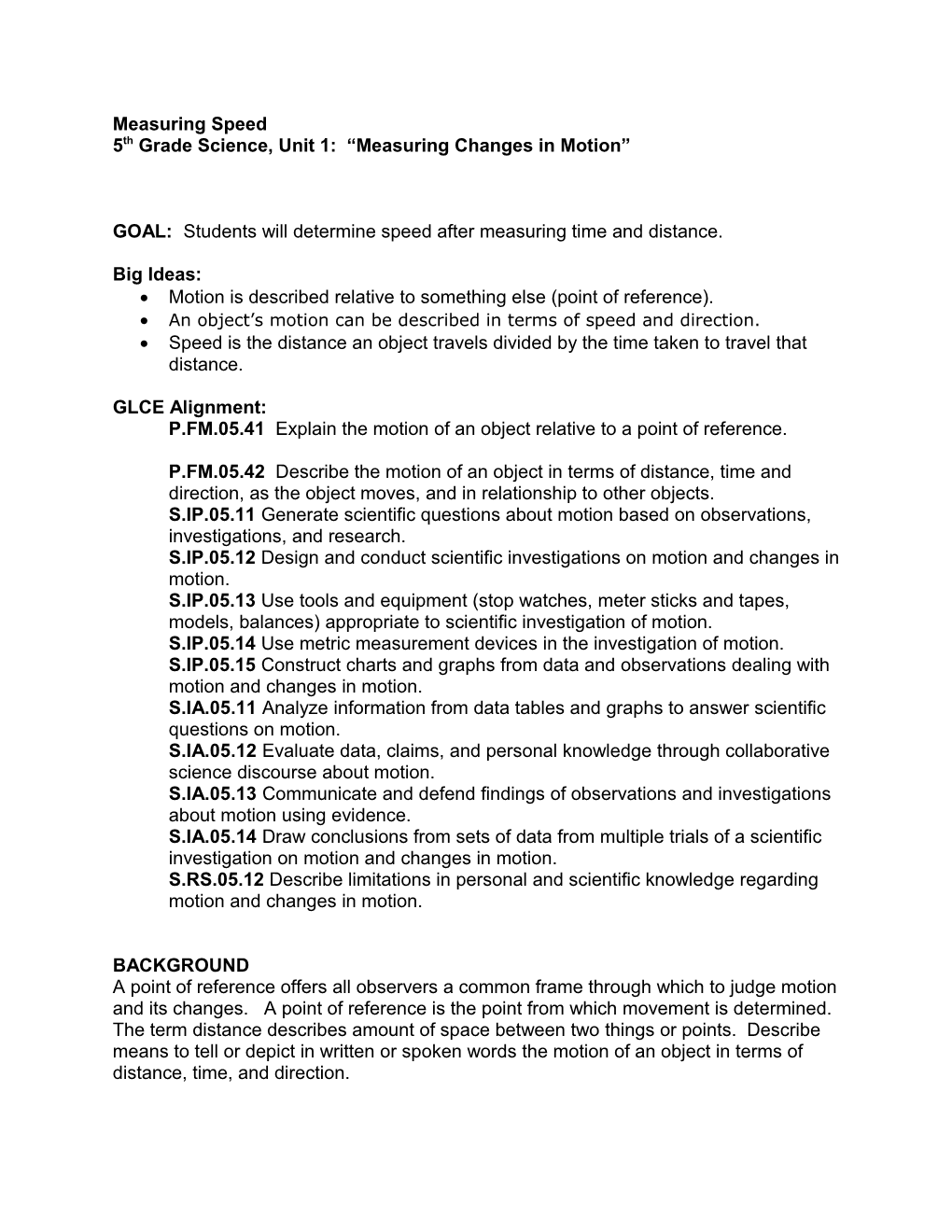# 5Th Grade Science, Unit 1: Measuring Changes in MotionMeasuring Speed

5th Grade Science, Unit 1: “Measuring Changes in Motion”

GOAL: Students will determine speed after measuring time and distance.

Big Ideas:

• Motion is described relative to something else (point of reference).
• An object’s motion can be described in terms of speed and direction.
• Speed is the distance an object travels divided by the time taken to travel that distance.

GLCE Alignment:

P.FM.05.41 Explain the motion of an object relative to a point of reference.

P.FM.05.42 Describe the motion of an object in terms of distance, time and direction, as the object moves, and in relationship to other objects.

S.IP.05.11 Generate scientific questions about motion based on observations, investigations, and research.

S.IP.05.12 Design and conduct scientific investigations on motion and changes in motion.

S.IP.05.13 Use tools and equipment (stop watches, meter sticks and tapes, models, balances) appropriate to scientific investigation of motion.

S.IP.05.14 Use metric measurement devices in the investigation of motion.

S.IP.05.15 Construct charts and graphs from data and observations dealing with motion and changes in motion.

S.IA.05.11 Analyze information from data tables and graphs to answer scientific questions on motion.

S.IA.05.12 Evaluate data, claims, and personal knowledge through collaborative science discourse about motion.

S.IA.05.13 Communicate and defend findings of observations and investigations about motion using evidence.

S.IA.05.14 Draw conclusions from sets of data from multiple trials of a scientific investigation on motion and changes in motion.

S.RS.05.12 Describe limitations in personal and scientific knowledge regarding motion and changes in motion.

BACKGROUND

A point of reference offers all observers a common frame through which to judge motion

and its changes. A point of reference is the point from which movement is determined.

The term distance describes amount of space between two things or points. Describe

means to tell or depict in written or spoken words the motion of an object in terms of

distance, time, and direction.

Time Frame of Activity:

1 50-minute session

VOCABULARY:

speed

distance

relative position

gravity

time interval

variable

MATERIALS

For the teacher:

1 car speed data table for presentation

For each group of four students:

1 board

1 book (the same thickness for all groups)

1 small toy car with moving wheels

1timer, stop watch, or watch with second hand

1 meterstick

1 calculator

For each student:

1 car speed data table

PROCEDURE:

A. TEACHING SUGGESTIONS

I. Introduce the concept of speed to the students.

Ask students to give examples of when measuring speed is important in “real life.” Ask them to give examples of units that measure speed (mph, km/h) as well as devices that measure speed (speedometer, radar gun).

Ask students how speed is measured? Tell students that two variables are important for measuring speed: the distance traveled and the time taken to travel this distance. Tell students that they will be measuring the speed of their toy cars in centimeters (distance) per second (time), cm/s.

After displaying the car speed recording sheet to the class, ask students why think there will be four trials in this activity. List the many possibilities for error (releasing the car at the same reference point, timing errors, etc.).

II. Do the activity: students measure and time the toy cars.

Students should be in groups of four.

Step 1: Distribute materials for the activity. Students set up the board, raising one end and placing it on the book. The meterstick is placed at the end of the board.

Step 2: A student releases the toy car from the top of the board.

Step 3: A student starts the timer/stopwatch as the car passes the beginning of the meterstick, stopping the timer/stopwatch as the car passes the end of the meterstick.

Step 4: Students calculate the time elapsed for the car to travel 100 centimeters, recording their calculations on the data table.

Step 5: Students calculate the speed for each trial, dividing the distance by the time. They record the speed for each trial on the sheet, averaging the results of the four trials.

III. Discuss the variables in the activity.

Each group should report its results. Students should recognize that “variables” are the “factors” in the experiment. The weight of the car was consistent, as was the distance; the time interval was not.

B. STUDENT MASTER

This is on the following page.

Car Speed Data Table and Worksheet

Trial / Distance (cm) / Time (s) / Speed (cm/s)
1 / 100
2 / 100
3 / 100
4 / 100

Average the speed:

Trial 1 + Trial 2 + Trial 3 + Trial 4 = TOTAL divided by 4 = Average speed

_____ + _____ + _____ + _____ = ______/ 4 = ______

What do you need to know to calculate the speed of a moving object?

______

What is the equation used to calculate speed?

______

If you skateboard 100 meters in 10 seconds, what is your speed?

______

What is the average speed of a person who walks 8 miles in 2 hours?

______

RESULTS and CONCLUSIONS

Students should report that most cars will take 1 to 2 seconds to travel the 100cm distance. Discuss the questions on the worksheet.